This post is a summary and paper skimming on rotation invariance and equivariance related research. So, this post will be keep updating by the time.

# Learning a rotation invariant detector with rotatable bounding box

• Conference: CVPR2018

## Summary

• Problem Statement
• Detection of arbitrarily rotated objects is a challenging task due to the difficulties of locating the multi-angle objects and separating them effectively from the background.
• The existing methods are not robust to angle varies of the objects because of the use of traditional bounding box, which is a rotation variant structure for locating rotated objects.
• Research Objective
• To suggest object detector which is rotation invariant
• Proposed Solution
• Propose rotatable bounding box (RBox) and detector (DRBox) which can handle the situation where the orientation angles of the objects are arbitrary.
• The training of DRBox forces the detection networks to learn the correct orientation angle of the objects, so that the rotation invariant property can be achieved.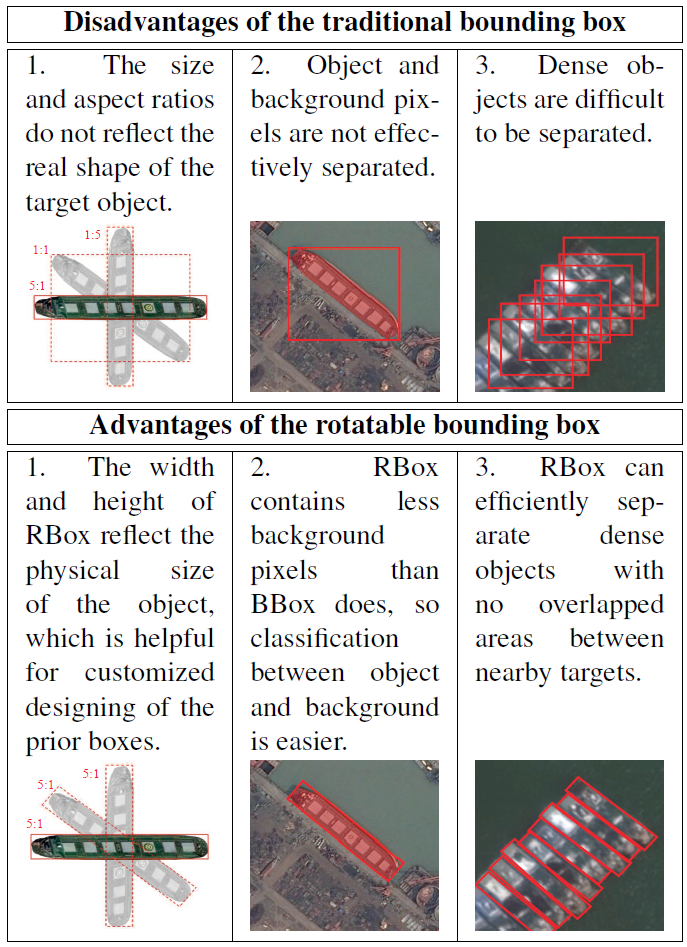Figure: Comparision of traditional bounding box and rotatable bounding box.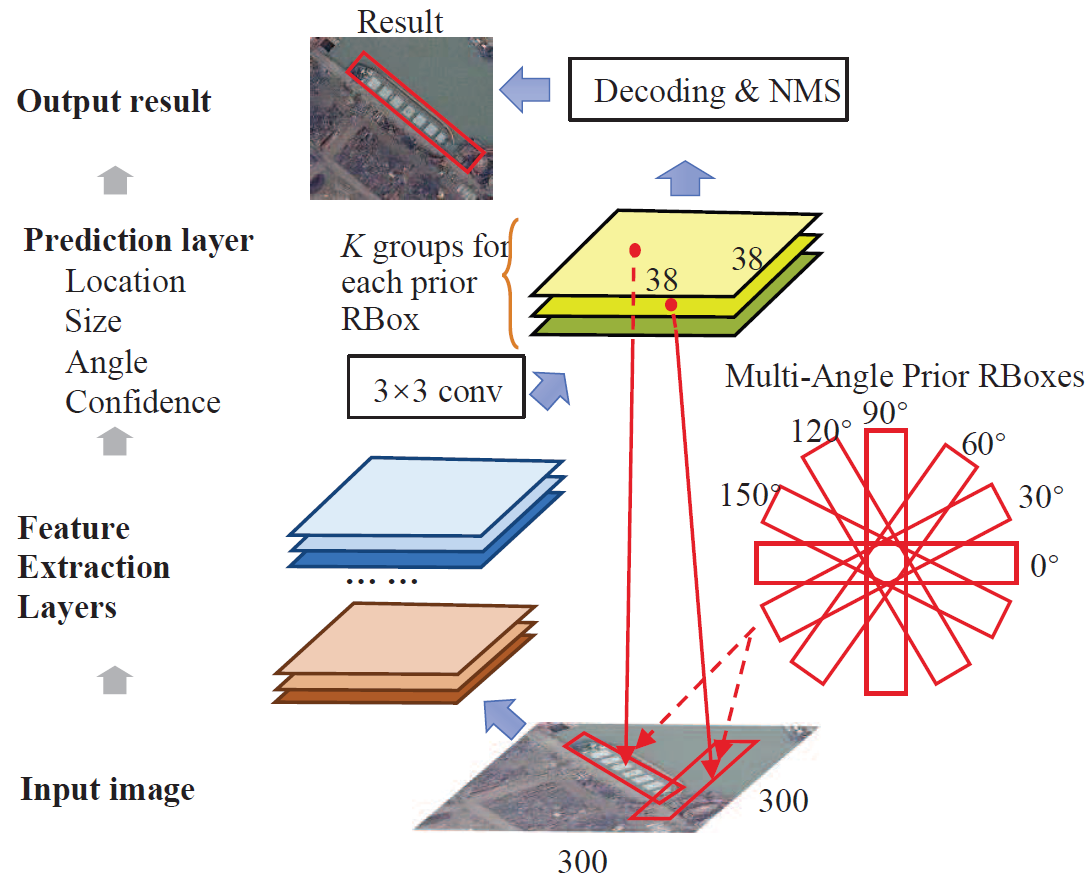Figure: The networks structure of DRBox. The networks structure of DRBox is similar with other box based methods except for the use of multi-angle prior RBoxes. DRBox searches for objects using sliding and rotation prior RBoxes on input image and then output locations of objects besides with their orientation angles.

• Contribution
• Compared with Faster R-CNN and CNN, DRBox performs much better than traditional bounding box based methods do on the given tasks, and is more robust against rotation of input image and target objects.
• DRBox correctly outputs the orientation angles of the objects.

# Oriented Response Networks

• Conference: CVPR2017

## Summary

• Problem Statement
• Deep Convolution Neural Networks (DCNNs) ability in handling significant local and global image rotations remains limited.
• Research Objective
• To suggest orientation invariant deep feature for DCNNs
• Proposed Solution
• Propose Active Rotating Filters (ARFs) that actively rotate during convolution and produce feature maps with location and orientation explicitly encoded.
• An ARF acts as a virtual filter bank containing the filter itself and its multiple unmaterialised rotated versions.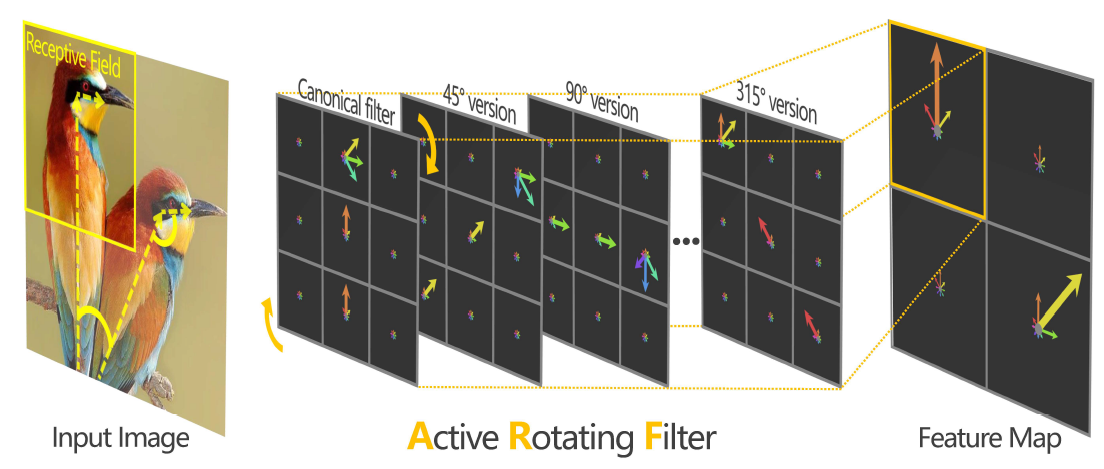Figure: An ARF is a filter of the size W X W X N, and viewed as N-directional points on a W X W grid. The form of the ARF enables it to effectively define relative rotations, e.g., the head rotation of a bird about its body. An ARF actively rotates during convolution; thus it acts as a virtual filter bank containing the canonical filter itself and its multiple unmaterialised rotated versions. In this example, the location and orientation of birds in different postures are captured by the ARF and explicitly encoded into a feature map.

• Contribution
• Specified Active Rotating Filters and Oriented Response Convolution, improved the most fundamental module of DCNN and endowed DCNN the capability of explicitly encoding hierarchical orientation information.
• Applied such orientation information to rotation-invariant image classification and object orientation estimation.
• Upgraded successful DCNNs including VGG, ResNet, TI-Pooling and STN to ORNs, achieving state-of-the-art performance with significantly fewer network parameters on popular benchmarks.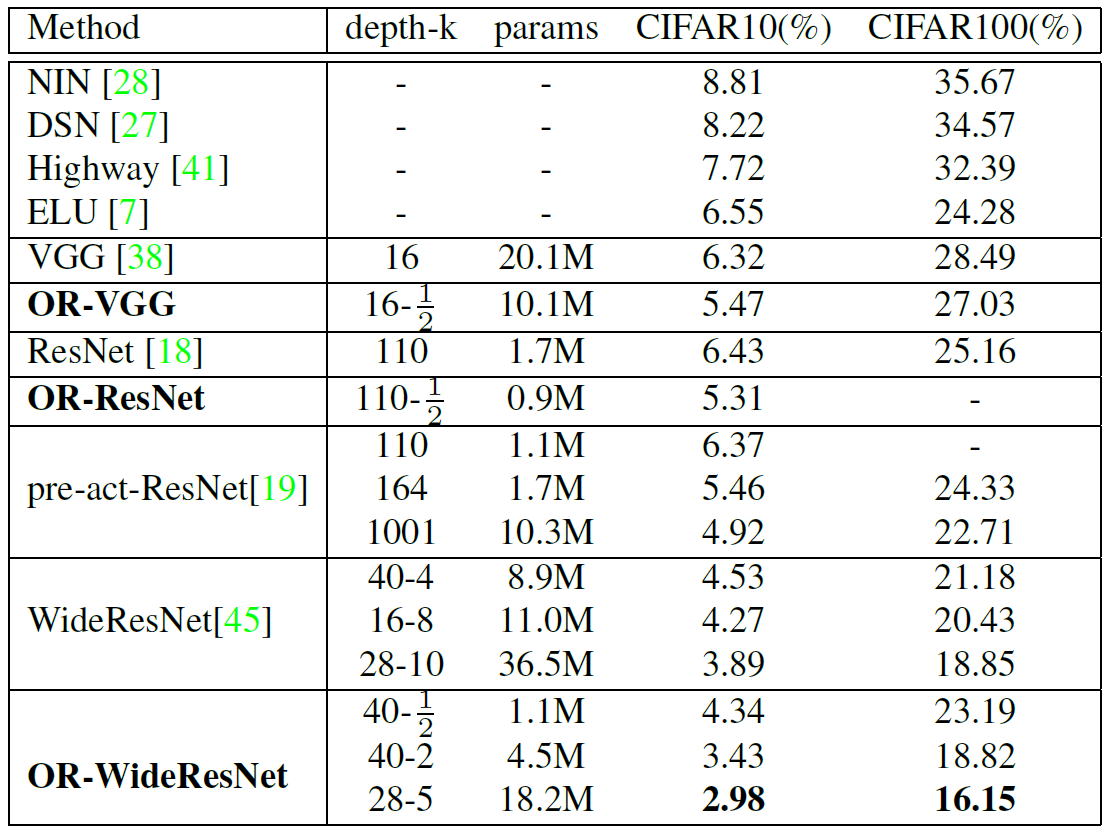Figure: Results on the natural image classification benchmark. In the second column, k is the widening factor corresponding to the number of filters in each layer.

# Unsupervised representation learning by predicting image rotations

• Conference: ICLR2018

## Summary

• Problem Statement
• In computer vision task, they usually require massive amounts of manually labeled data, which is both expensive and impractical to scale.
• Therefore, unsupervised semantic feature learning is important.
• Research Objective
• To learn ConvNet based semantic features in an unsupervised manner.
• Proposed Solution
• Propose to learn image features by training ConvNets to recognize the 2d rotation that is applied to the image that it gets as input.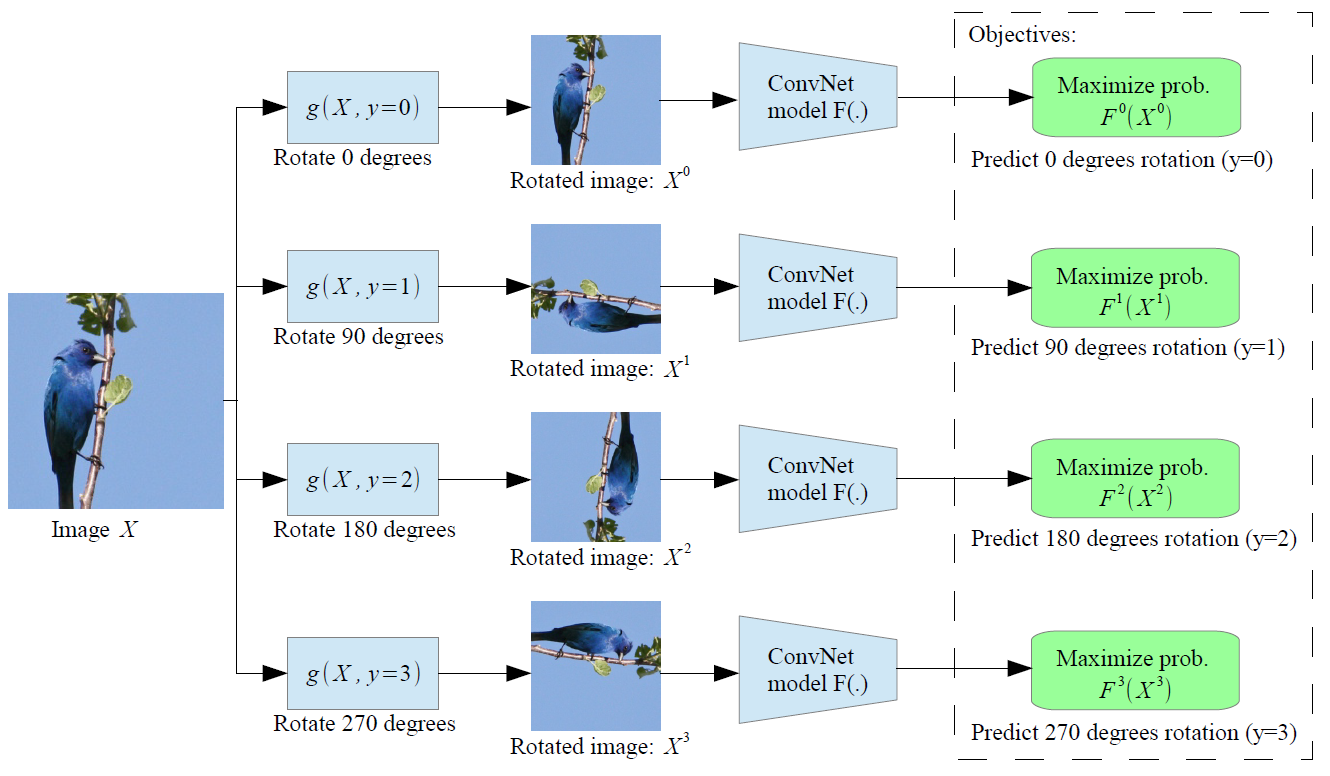Figure: Proposed self-supervised task. Given four possible geometric transformations, the 0, 90, 180, 270 degrees rotations, proposed method train a ConvNet model $F(.)$ to recognize the rotation that is applied to the image that it gets as input. $F^y(X^{y^*})$ is the probability of rotation transformation $y$ predicted by model $F(.)$ when it gets as input an image that has been transformed by the rotation transformation $y^*$.

• Contribution
• Offers a powerful supervisory signal for semantic feature learning
• In all of various evaluation, proposed self-supervised formulation demonstrates state-of-the-art results with dramatic improvements w.r.t. prior unsupervised approaches.
• Proposed self-supervised learning approach significantly narrows the gap between unsupervised and supervised feature learning.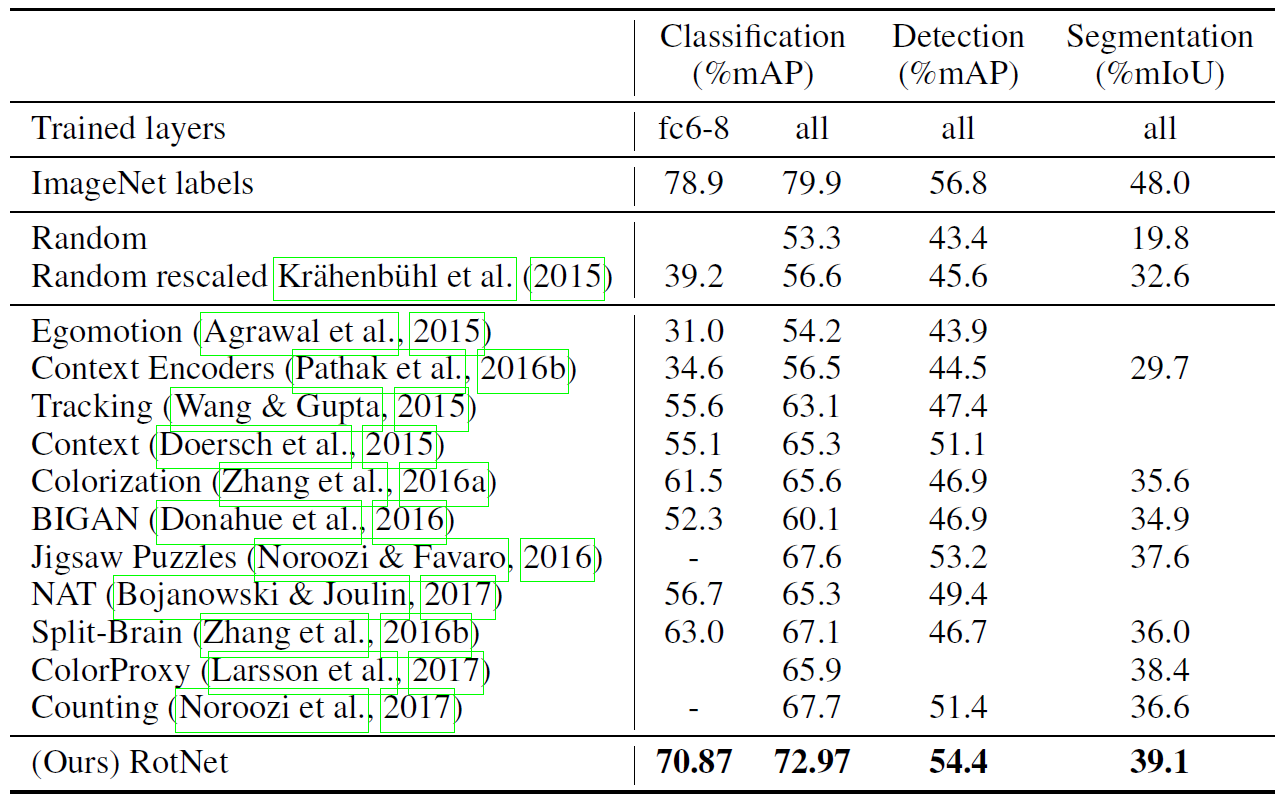Figure: Task & Dataset Generalization: PASCAL VOC 2007 classification and detection results, and PASCAL VOC 2012 segmentation results.

# Arbitrary-oriented scene text detection via rotation proposals

• Conference: IEEE Transactions on Multimedia 2018

## Summary

• Problem Statement
• In real-world applications, a large number of the text regions are not horizontal.
• Thus, recent years’ horizontal-specific methods cannot be widely applied in practice.
• Research Objective
• To detect arbitrary-oriented text in natural scene images
• Proposed Solution
• Propose the Rotation Region Proposal Networks (RRPN), which are designed to generate inclined proposals with text orientation angle information.
• The angle information is then adapted for bounding box regression to make the proposals more accurately fit into the text region in terms of the orientation.
• The Rotation Region-of-Interest (RRoI) pooling layer is proposed to project arbitrary-oriented proposals to a feature map for a text region classifier.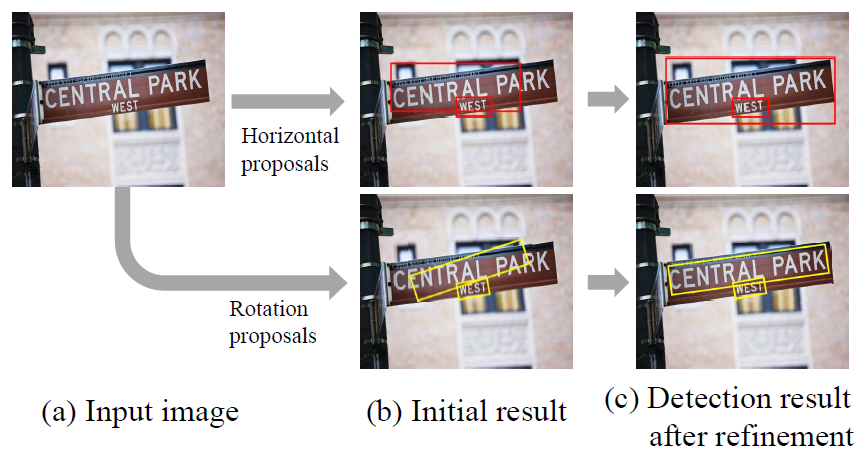Figure: First row: text detection based on horizontal bounding box proposal and bounding box regression of Faster-RCNN. Second row: detection using rotation region proposal and bounding box regression with orientation step.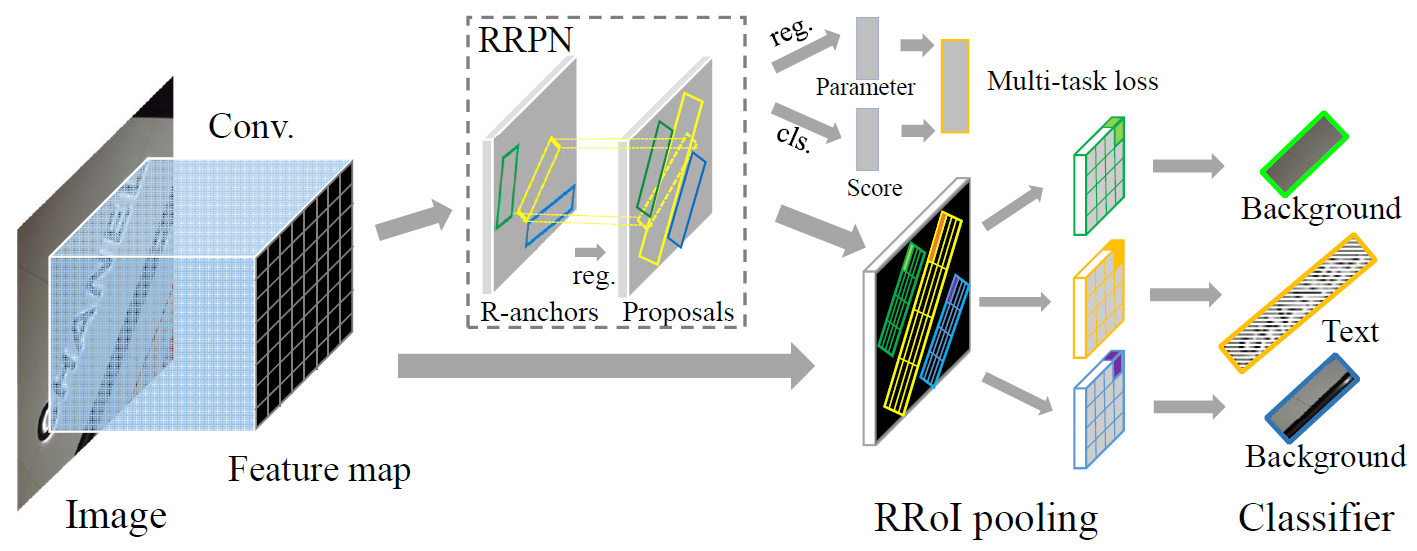Figure: Rotation-based text detection pipeline.

• Contribution
• Proposed solution has the ability to predict the orientation of a text line using a region-proposal-based approach; thus, the proposals can better fit the text region, and the ranged text region can be easily rectified and is more convenient for text reading.
• RRoI pooling layer and learning of the rotated proposal are incorporated into the region-proposal-based architecture, which ensures the computational efficiency of text detection compared with segmentation-based text detection systems.
• Propose strategies for the refinement of region proposals with arbitrary orientation to improve the performance of arbitrary-oriented text detection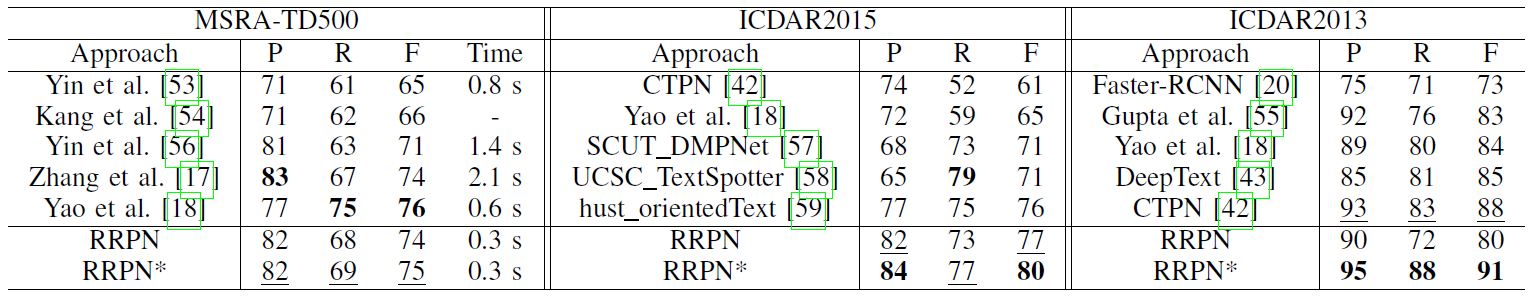Figure: Comparison with state-of-the-art approaches on three benchmarks. Bold text denotes the top result, while underlined text corresponds to the second runner-up.

# [Blog] Correcting Image Orientation Using Convolutional Neural Networks

• Objective
• To predict the rotation angle of an image and correct it into upright image
• Network
• Used CNN model
• Simple CNN for MNIST
• ResNet50 with pre-trained on ImageNet for Google Street View
• The output: we need the network to predict the image’s rotation angle, which can then be used to rotate the image in the opposite direction to correct its orientation.
• Used classification: the network should produce a vector of 360 values instead of a single value. Each value of that vector represents the probability between 0 and 1 of each class being the correct one.
• The author mentioned that using classification showed better performance than regression.
• Used angle_error as a metric: to monitor the accuracy of the model during training. It will be in charge of periodically computing the angle difference between predicted angles and true angles.
• Used Keras based on TensorFlow
• Data set
• MNIST and Google Street View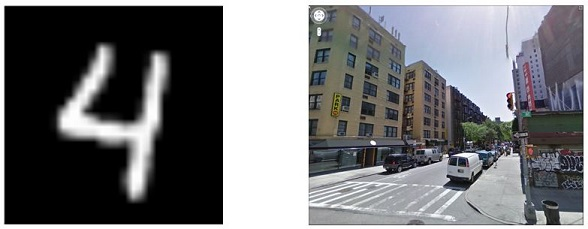• RotNet on MNIST
• After 50 epochs, the network achieves an average angle error of 6-7 degrees in the validation set.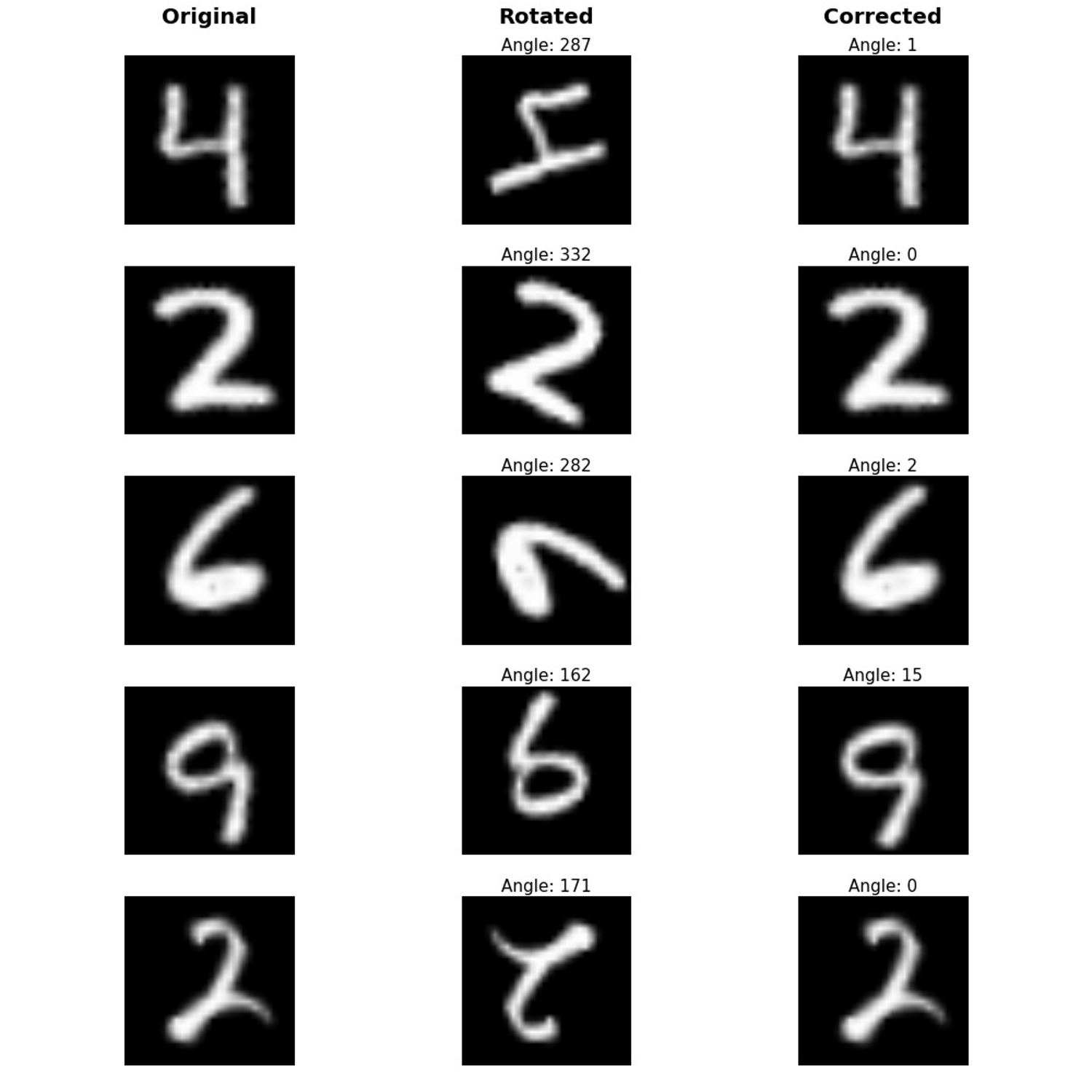• RotNet on Google Street View
• After 10 epochs to get an average angle error of 1-2 degrees.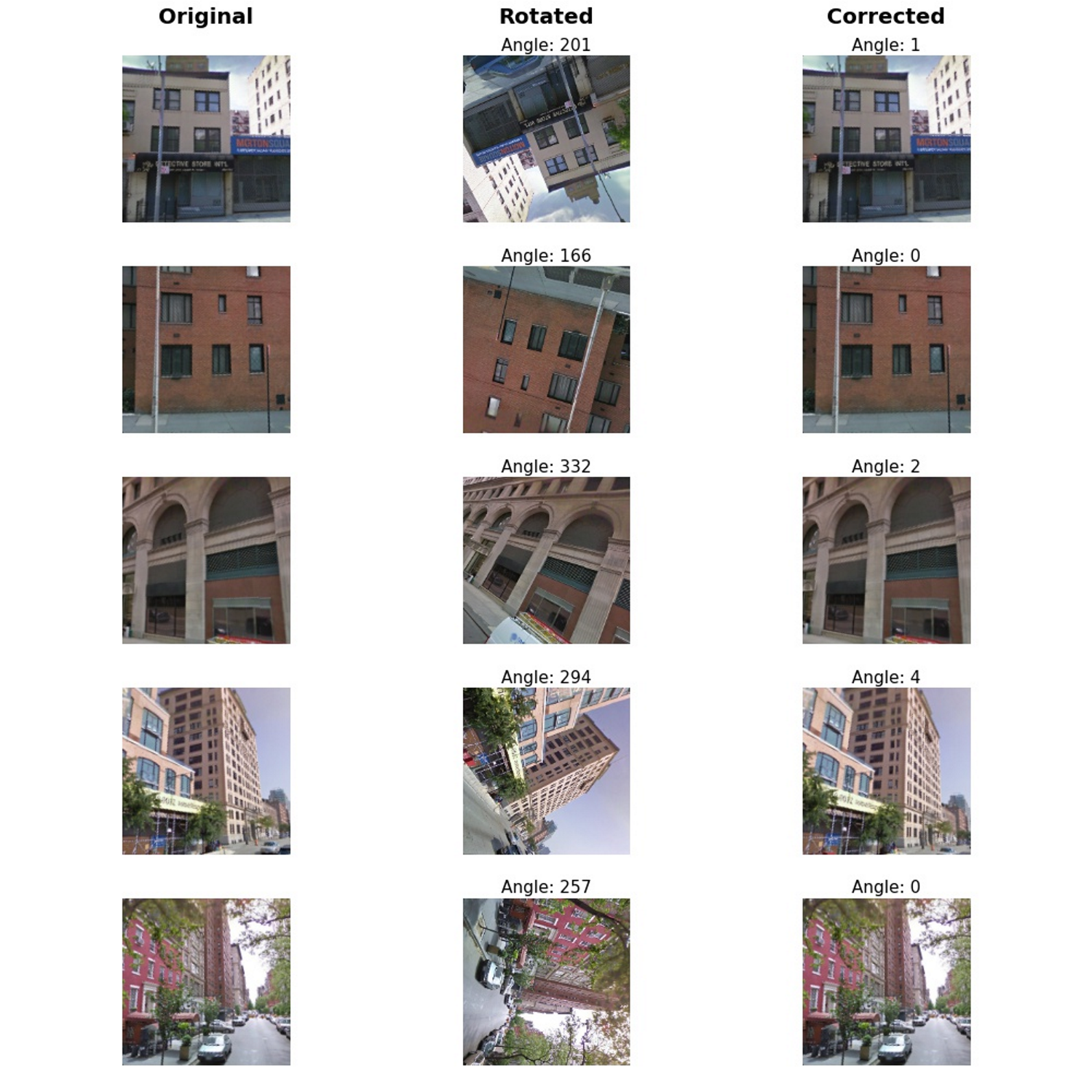# Deep rotation equivariant network

• Conference: Neurocomputing 2018

## Summary

• Problem Statement
• To learn rotation equivariance, feature maps should be copied and rotated four times in each layer which causes much running time and memory overhead.
• Research Objective
• To suggest a network which is rotation equivariant and efficient on speed and memory usage.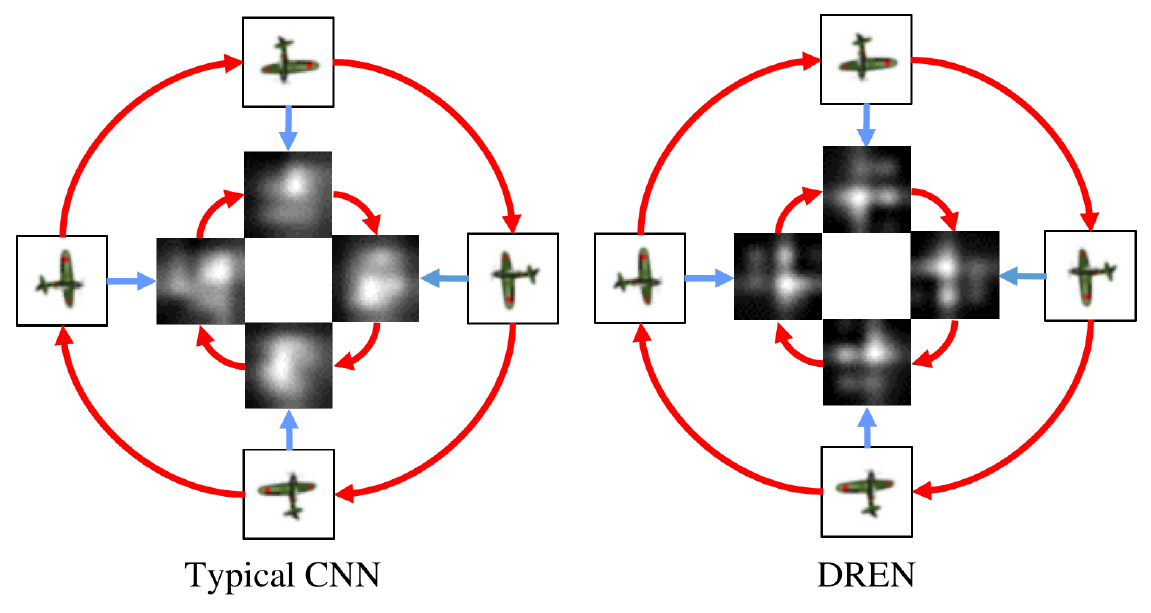Figure: Latent representations learnt by a CNN and a DREN. Features produced by a DREN is equivariant to rotation while that produced by a typical CNN is not.

• Proposed Solution
• Propose the Deep Rotation Equivariant Network consisting of cycle layers, isotonic layers and decycle layers.
• Proposed layers apply rotation transformation on filters rather than feature maps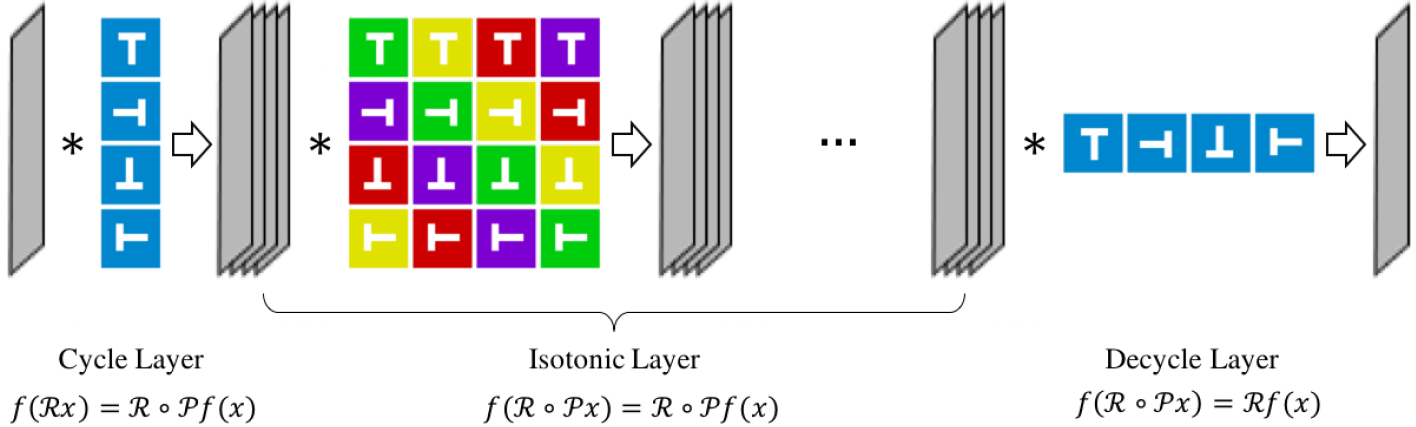Figure: The framework of Deep Rotation Equivariant Network. The gray panels represent input, feature maps and output. Each square represents a weight kernel. The letter ’T’ is utilized for distinguishing orientation clearly. The different colors of kernel background indicate that the kernel are not qualitatively equivalent. Although this figure seems similar to that one in this paper, there are 3 critical differences: 1. We apply rotation on the filters rather than the feature maps. 2. The matrix in an isotonic layer is different in order from the matrix of cyclic rolling operation in this paper. 3. The decycle layer is a special convolution layer, different from the cyclic pooling applied in this paper.

• Contribution
• Evaluate DRENs on Rotated MNIST and CIFAR-10 datasets and demonstrate that it can improve the performance of state-of-the-art.
• Achieve a speed up of more than 2 times with even less memory overhead.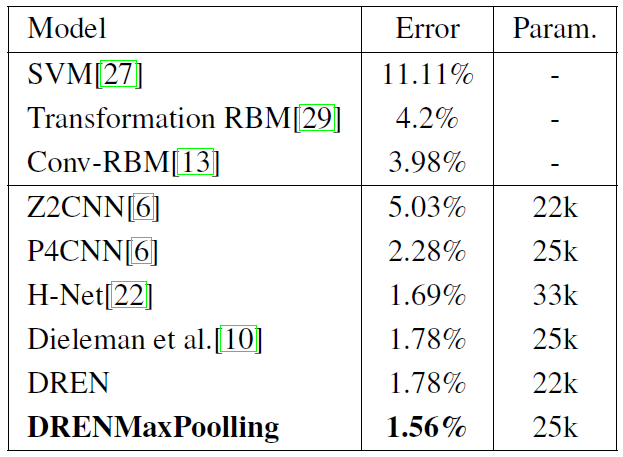Figure: Performance of various models on Rotated MNIST.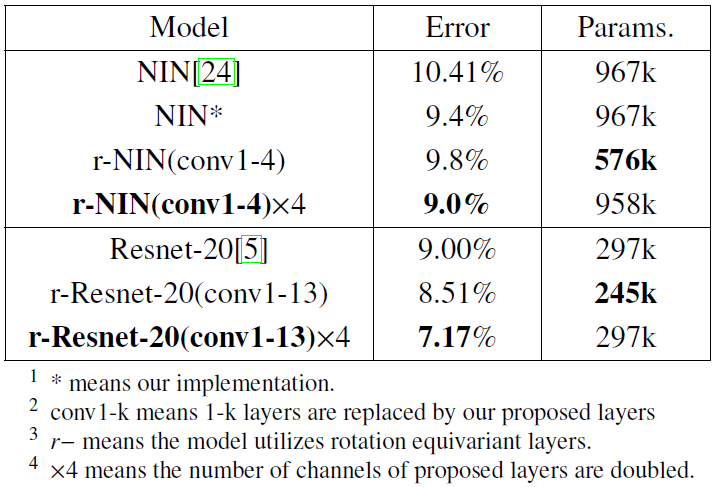Figure: Performance of various models on CIFAR-10.

# Self-supervised learning of geometrically stable features through probabilistic introspection

• Conference: CVPR 2018

## Summary

• Problem Statement
• While several authors have looked at self-supervision for tasks such as image classification and segmentation, less work has been done on tasks that involve understanding the geometric properties of object categories.
• Research Objective
• Aim at extending it to geometry-oriented tasks such as semantic matching and part detection.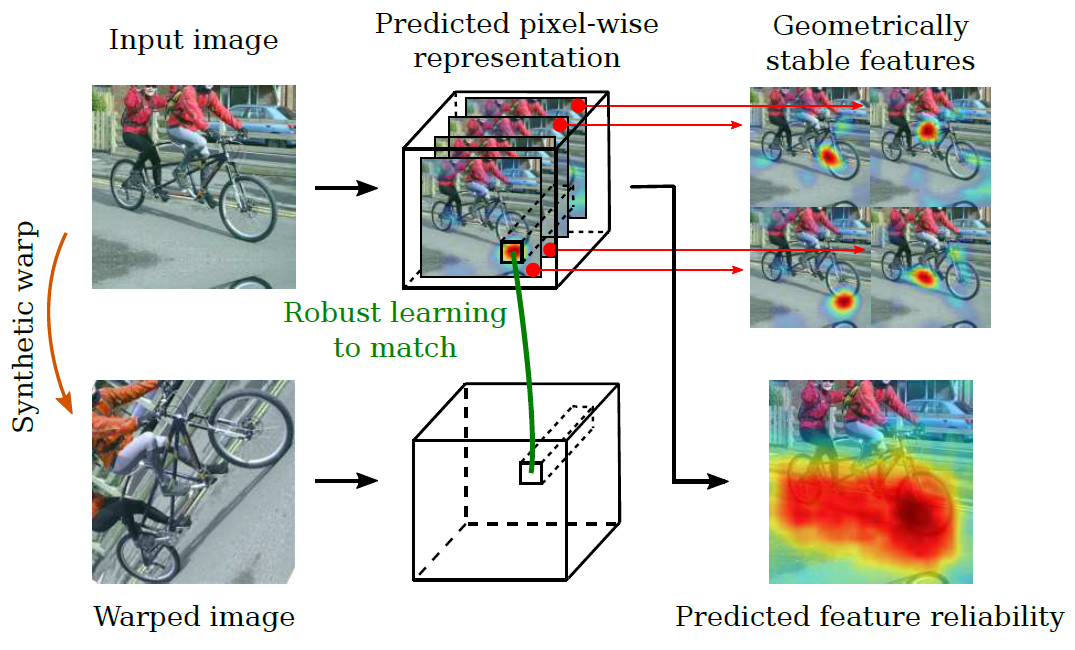Figure: Proposed approach leverages correspondences obtained from synthetic warps in order to self-supervise the learning of a dense image representation. This results in highly localized and geometrically stable features. The use of a novel robust probabilistic formulation allows to additionally predict a pixel-level confidence map that estimates the matching ability of these features.

• Proposed Solution
• Proposed approach learns dense distinctive visual descriptors from an unlabeled dataset of images using synthetic image transformations.
• It does so by means of a robust probabilistic formulation that can introspectively determine which image regions are likely to result in stable image matching.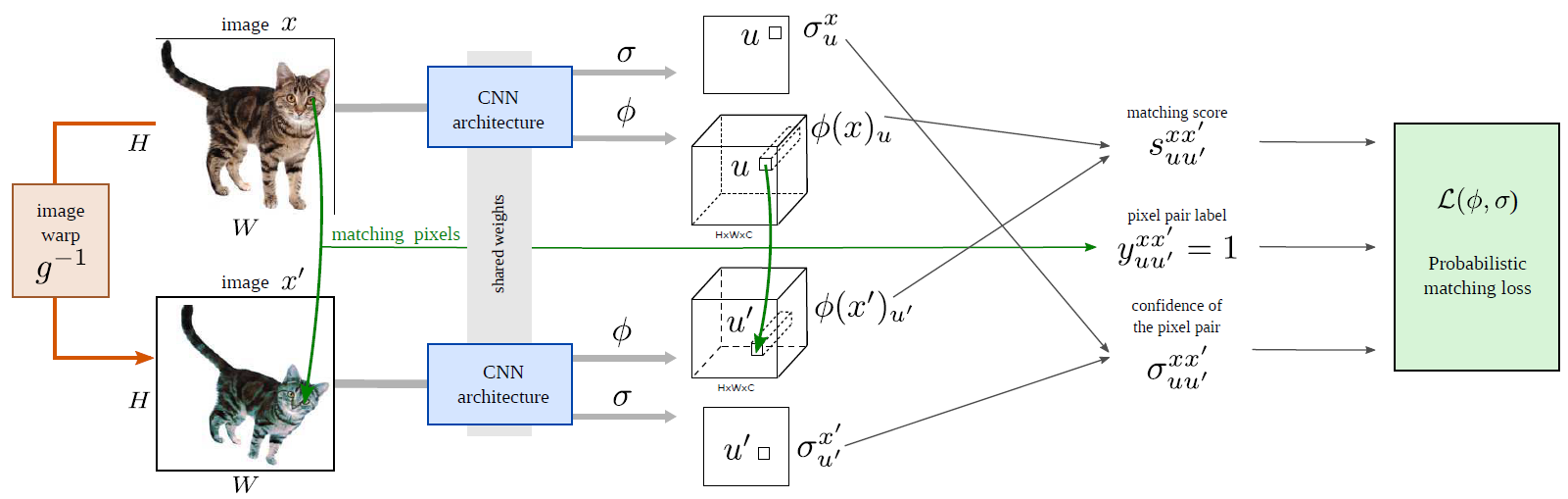Figure: Overview of our approach. Image $x$ is warped into image $x′$ using the transformation $g^{−1}$. Pairs of pixels and their labels (encoding whether they match or not according to $g^{−1}$) are used together with a probabilistic matching loss to train our architecture that predicts i) a dense image feature $φ(x)$ and ii) a pixel level confidence value $σ(x)$.

• Contribution
• A network pre-trained in this manner requires significantly less supervision to learn semantic object parts compared to numerous pre-training alternatives.
• The pre-trained representation is excellent for semantic object matching.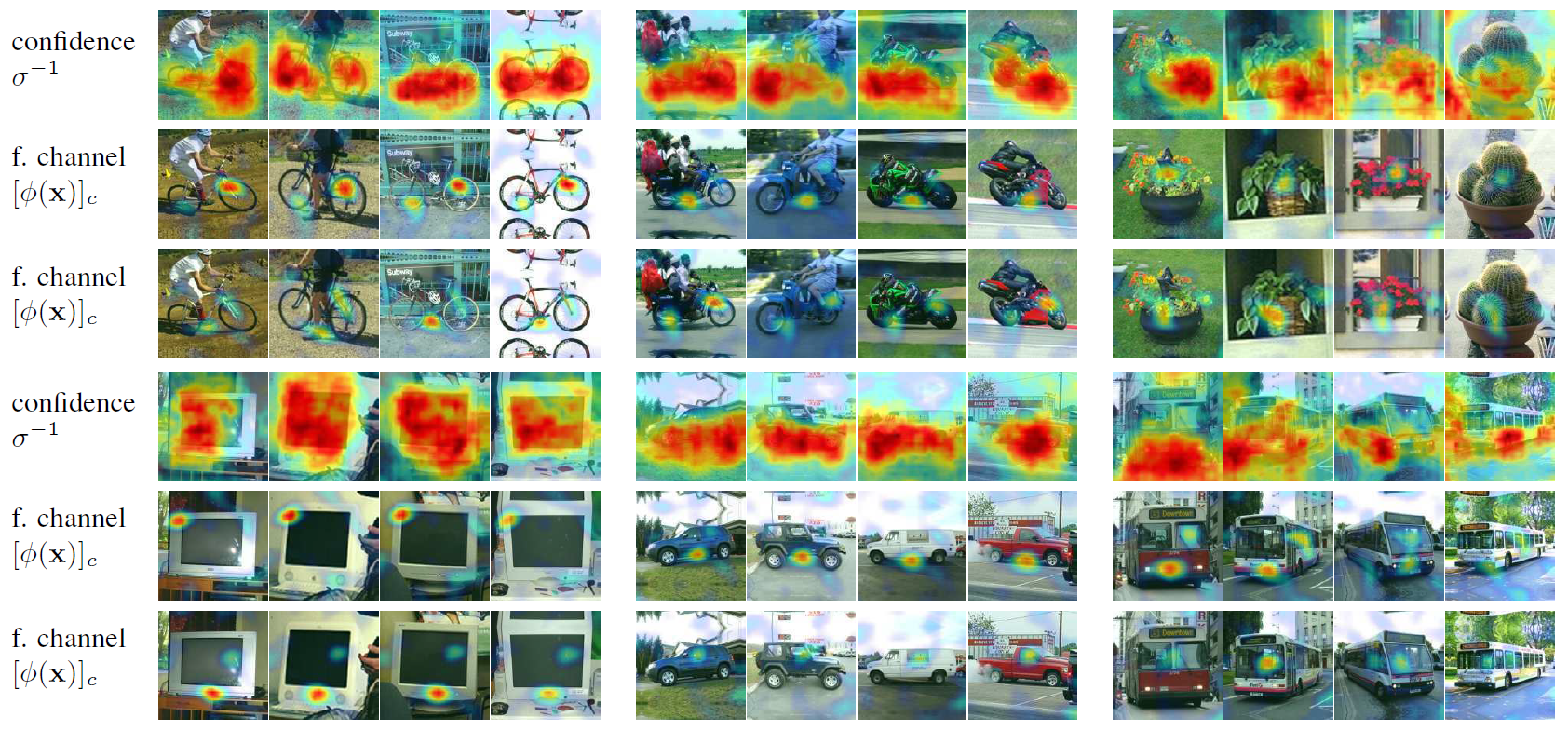Figure: Qualitative analysis of the learned equivariant feature representation $φ$ visualizing predicted confidence maps $σ^{−1}$ and several responses max($[φ(x)]_c, 0$) of different channels $c$ of the representation, for six different categories.

# Real-time rotation-invariant face detection

• Title: Real-time rotation-invariant face detection with progressive calibration networks
• Conference: CVPR 2018

## Summary

• Problem Statement
• Rotation-invariant face detection is widelyrequired in unconstrained applications but still remains as a challenging task, due to the large variations of face appearances.
• Most existing methods compromise with speed or accuracy to handle the large rotation-in-plane (RIP) variations.
• Research Objective
• To perform rotation-invariant face detection
• Proposed Solution
• Propose Progressive Calibration Networks (PCN) to perform rotation-invariant face detection in a coarse-to-fine manner
• PCN consists of three stages, each of which not only distinguishes the faces from non-faces, but also calibrates the RIP orientation of each face candidate to upright progressively.
• By dividing the calibration process into several progressive steps and only predicting coarse orientations in early stages, PCN can achieve precise and fast calibration.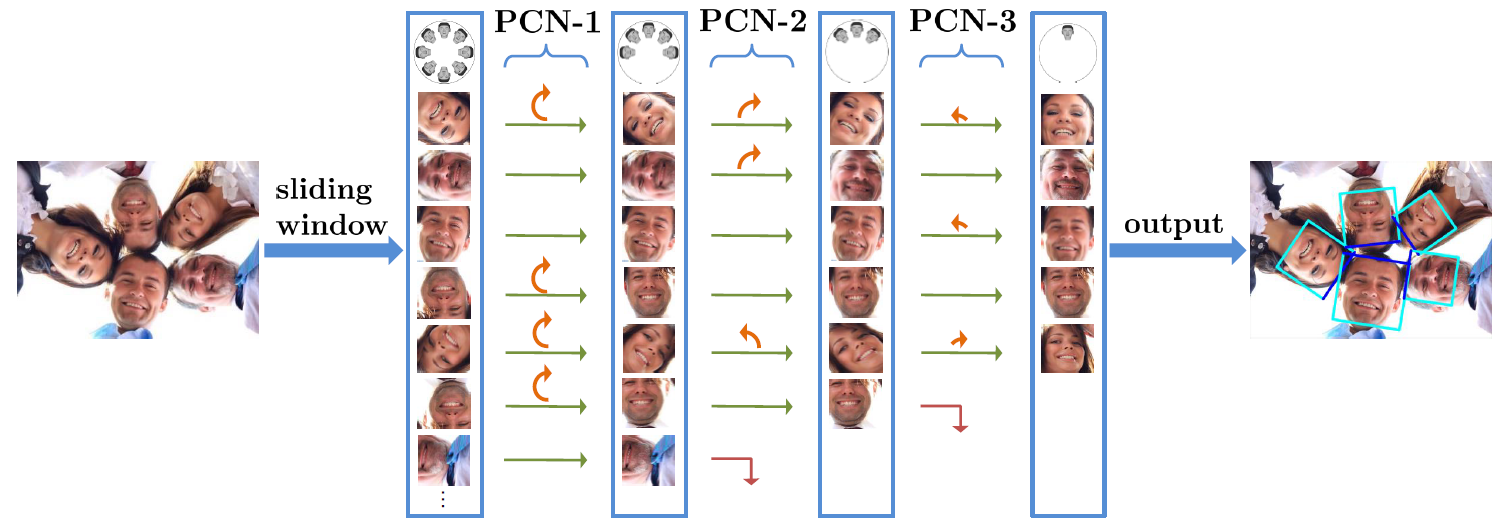Figure: An overview of our proposed progressive calibration networks (PCN) for rotation-invariant face detection. Our PCN progressively calibrates the RIP orientation of each face candidate to upright for better distinguishing faces from non-faces. Specifically, PCN-1 first identifies face candidates and calibrates those facing down to facing up, halving the range of RIP angles from [-180º, 180º] to [-90º, 90º]. Then the rotated face candidates are further distinguished and calibrated to an upright range of [-45º, 45º] in PCN-2, shrinking the RIP ranges by half again. Finally, PCN-3 makes the accurate final decision for each face candidate to determine whether it is a face and predict the precise RIP angle.

• Contribution
• By performing binary classification of face vs. non-face with gradually decreasing RIP ranges, PCN can accurately detect faces with full 360 RIP angles
• Such designs lead to a real-time rotation-invariant face detector.
• The experiments on multi-oriented FDDB and a challenging subset of WIDER FACE containing rotated faces in the wild show that proposed PCN achieves promising performance.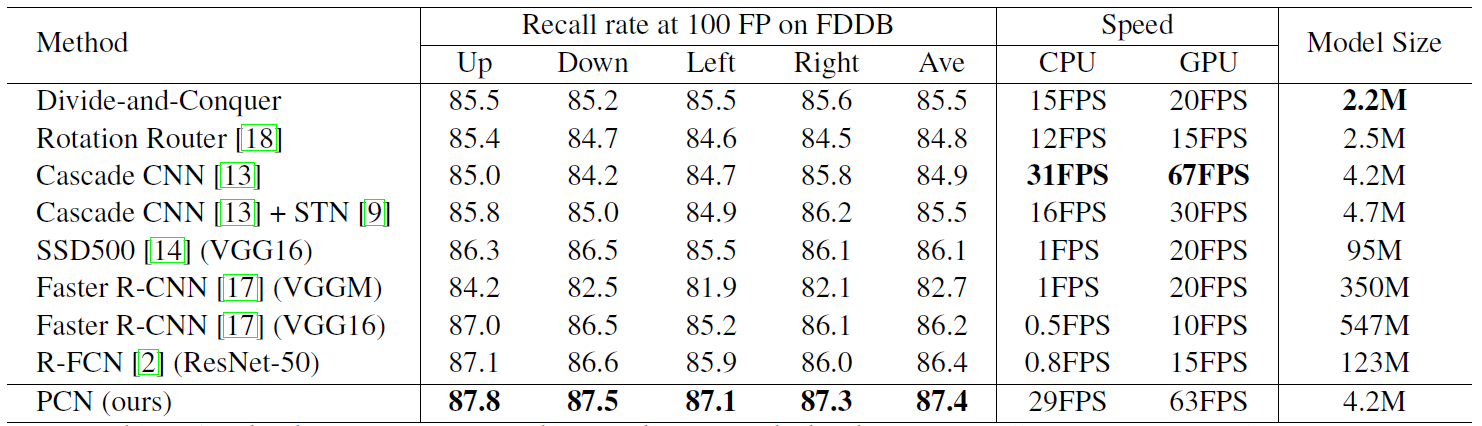Figure: Speed and accuracy comparison between different methods. The FDDB recall rate (%) is at 100 false positives.

# R2CNN

• Title: R2CNN: Rotational Region CNN for Orientation Robust Scene Text Detection

## Research Objective

• To detect arbitrary-oriented texts in natural scene images

## Proposed Solution

• Propose a method called Rotational Region CNN ($R^2CNN$)
• The framework is based on Faster R-CNN architecture.
• First, it uses the Region Proposal Network (RPN) to generate axis-aligned bounding boxes that enclose the texts with different orientations.
• Second, for each axis-aligned text box proposed by RPN, we extract its pooled features with different pooled sizes and the concatenated features are used to simultaneously predict the text/non-text score, axis-aligned box and inclined minimum area box.
• Proposed method use the coordinates of the first two points in clockwise and the height of the bounding box to represent and inclined rectangle ($x_1, y_1, x_2, y_2, h$).
• At last, it uses an inclined non-maximum suppression to get the detection results.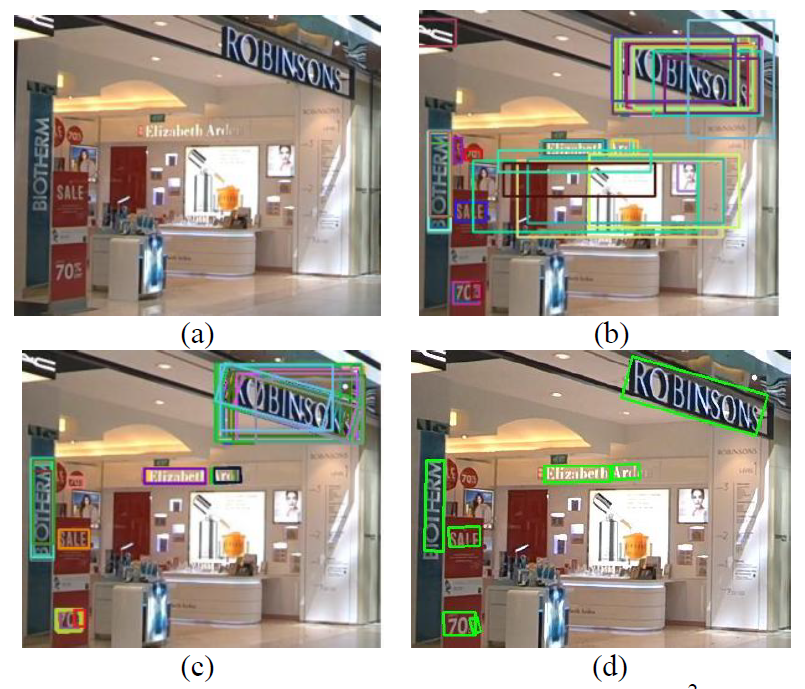Figure: The procedure of the proposed method R2CNN. (a) Original input image; (b) text regions (axis-aligned bounding boxes) generated by RPN; (c) predicted axis-aligned boxes and inclined minimum area boxes (each inclined box is associated with an axis-aligned box, and the associated box pair is indicated by the same color); (d) detection result after inclined non-maximum suppression.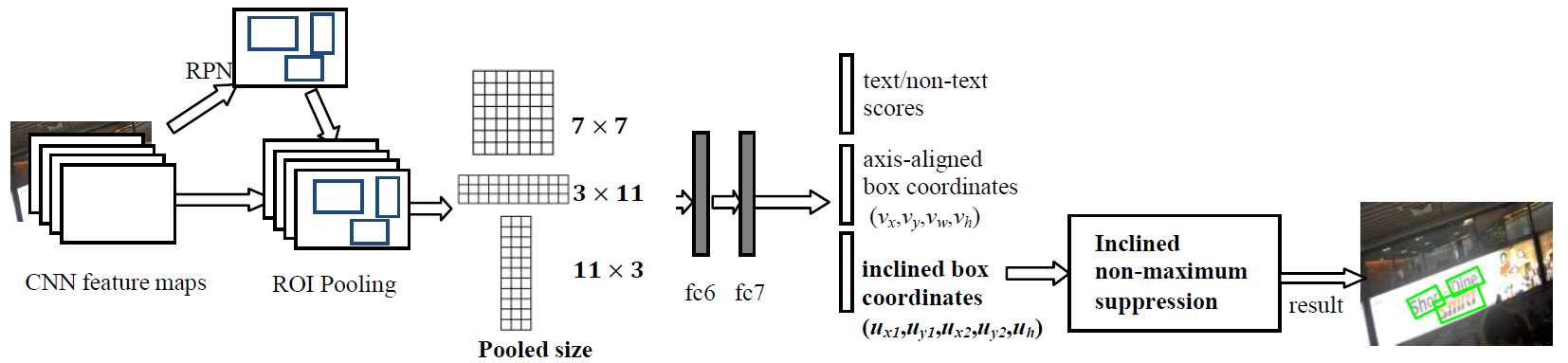Figure: The network architecture of Rotational Region CNN (R2CNN). The RPN is used for proposing axis-aligned bounding boxes that enclose the arbitrary-oriented texts. For each box generated by RPN, three ROIPoolings with different pooled sizes are performed and the pooled features are concatenated for predicting the text scores, axis-aligned box ($v_x,v_y,v_w,v_h$) and inclined minimum area box ($u_{x1},u_{y1},u_{x2},u_{y2},u_h$). Then an inclined non-maximum suppression is conducted on the inclined boxes to get the final result.

## Contribution

• Introduce a novel framework for detecting scene texts of arbitrary orientations.
• The arbitrary-oriented text detection problem is formulated as a multi-task problem. The core of the approach is predicting text scores, axis-aligned boxes and inclined minimum area boxes for each proposal generated by the RPN.
• To make the most of text characteristics, they do several ROIPoolings with different pooled sizes for each RPN proposal.
• Modification of Faster R-CNN also include adding a small anchor for detecting small scene texts and using inclined non-maximum suppression to post-process the detection candidates to get the final result.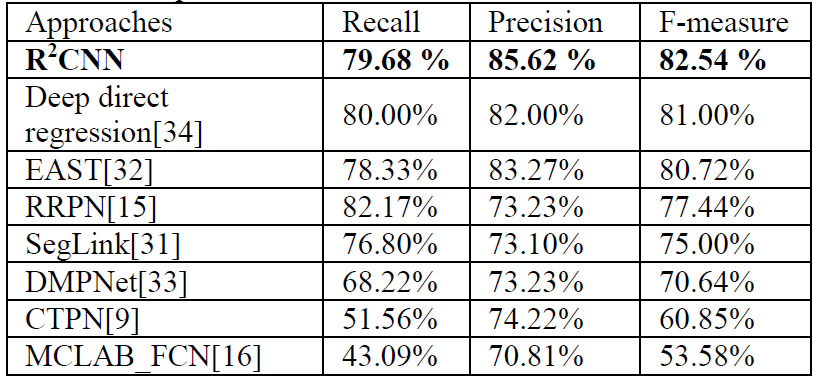Figure: Comparison with state-of-the-art on ICDAR2015.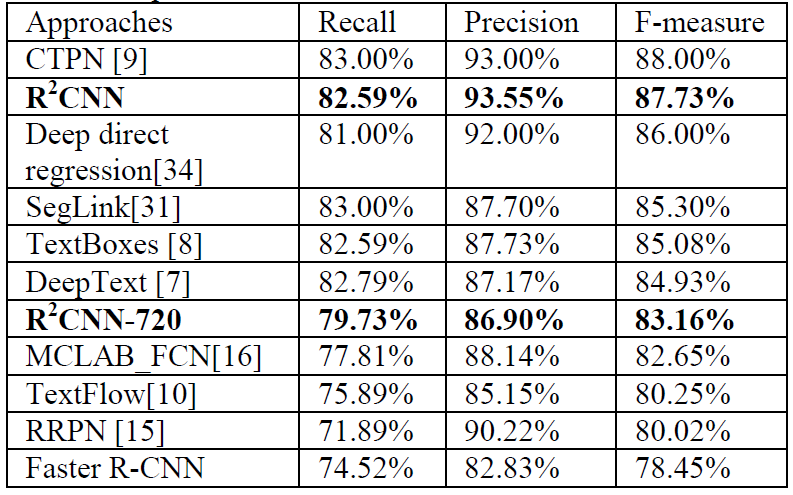Figure: Comparison with state-of-the-art on ICDAR2013.